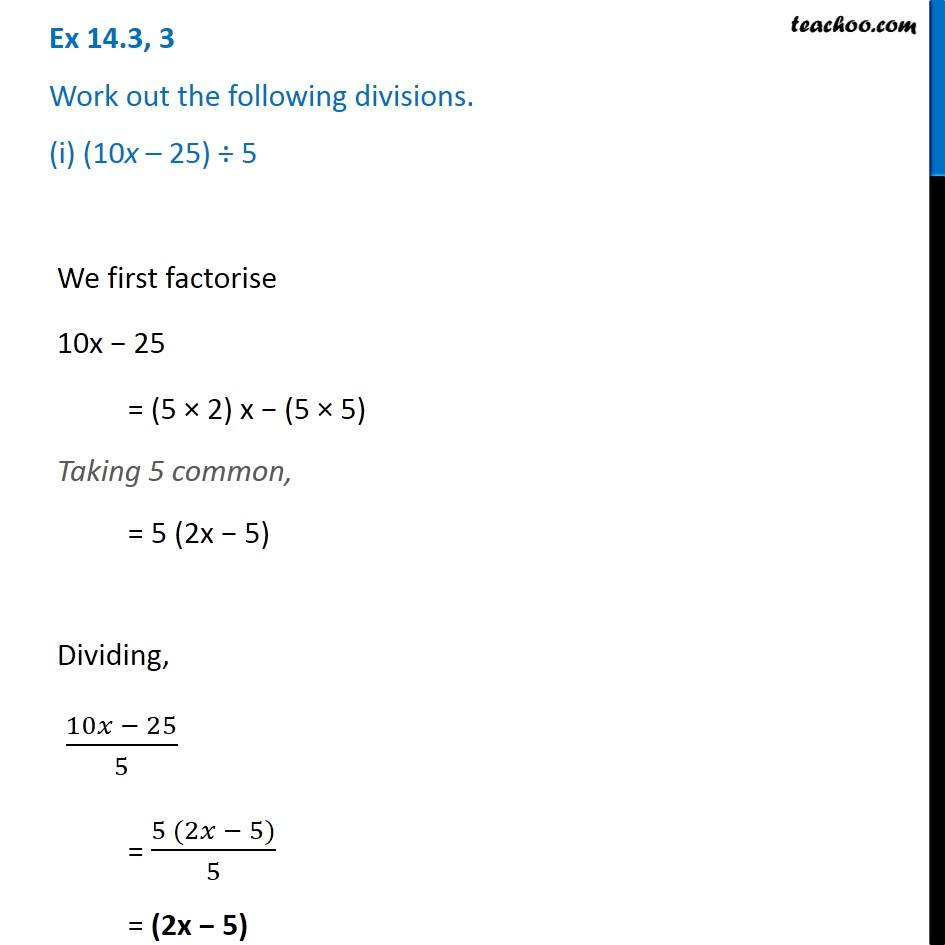1. Chapter 14 Class 8 Factorisation
2. Concept wise
3. Dividing two polynomials

Transcript

Ex 14.3, 3 Work out the following divisions. (i) (10x – 25) ÷ 5 We first factorise 10x − 25 = (5 × 2) x − (5 × 5) Taking 5 common, = 5 (2x − 5) Dividing, (10𝑥 − 25)/5 = (5 (2𝑥 − 5))/5 = (2x − 5)

Dividing two polynomials